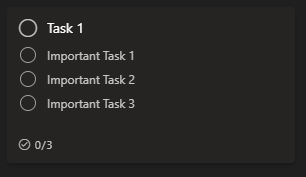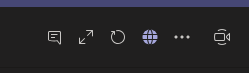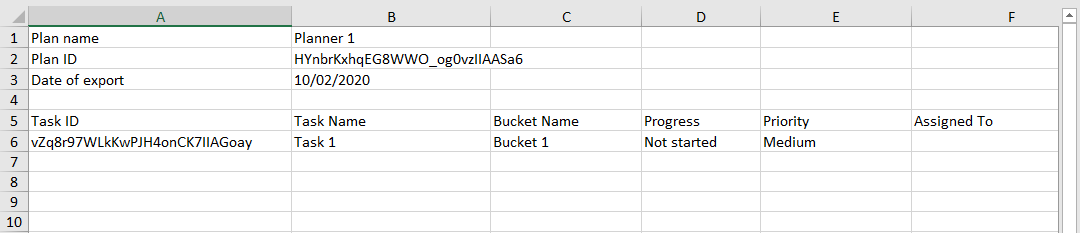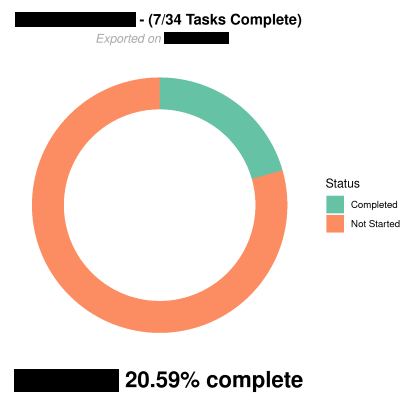Update: Since I made this post, I started development on a R package that aims to make what’s in this post extensible and easy to use. You can view it’s GitHub repo here or the documentation here.

Recently I came across the issue of trying to provide better visual statistics of project tasks. The problem with this was that the tasks were being tracked with Microsoft Planners in Teams using checklists as the primary indicator for task completion within each task in a bucket, like so:If you’re familiar with Planner, then you know that the built-in dashboard doesn’t account for checklists at all. If you’re not familiar, then you should know that Planner will consider this as one task – which makes sense in most cases. However, I wanted to account for the checklist. This is where R comes in.

## What is R?⌗

R is a programming language primarily used for statistical computing and graphics. I recently became familiar with it in a GIS class I took at UCSB, and decided I would put it to use for displaying dashboard-like plots. To do this, there one significant package that will help to accomplish this: `ggplot2`. You can read about it more here, but it’s essentially a package that provides pretty data visualization.

`ggplot2` is a part of the `tidyverse` package which includes a bunch of other packages for making data organization easier. To install it, all you need to do is run the command `install.packages("tidyverse")` in an R console. Alternatively, you can install the specific packages I’ll be using with:

`install.packages(c("ggplot2", "dplyr", "readxl", "stringr")`

## Getting Planner Data⌗

The nice thing about Planner is that it allows you to export the Planner as an Excel spreadsheet, which works wonderfully with R.

### From Teams⌗

If you’re using Teams primarily, you need to access the Planner from the web app to export. To do this, go to the tab for your Planner and in the top-right corner, click on the globe (Go to website).### On the Web App⌗

Once you’re on the Planner web app, open the full menu by clicking on the . . . next to the Charts and Schedule buttons, and clicking Export plan to Excel. Now you should have an excel spreadsheet for your planner.There’s a lot of different columns in this spreadsheet, but in this case we mostly want to focus on the Completed Checklist Items (This should be the O column.)

## Importing and Tidying Data in R⌗

Now that we have the Planner data in spreadsheet form, we need to import it into R and start working with it. To do this, we need a package: `readxl`. If you have the `tidyverse` package installed, `readxl` comes with it.

From this point, I’m going to assume you’re familiar with R. If you’re not, I suggest getting familiar with it by reading the Introduction to R Manual from the R-Project website. Anyways, back to working with the spreadsheet.

You might notice that the first 4 rows of the Planner data spreadsheet include some information that doesn’t allow us to directly import to R natively (as it wouldn’t create the correct tibble.) So, what we’re going to do is take the information that we need and remove the rows.

I do this fairly primitvely, but it works:

``````
library(tidyverse)

plan_name     <- "Planner Name" # Change this, or use colnames(plan_data[])
plan_date     <- plan_data[]
filtered_data <- setNames(plan_data, plan_data[4, ])
filtered_data <- filtered_data[-c(1,2,3,4), ]
# You can filter Buckets/Tasks out by using dplyr::filter()
#
# filtered_data <- filtered_data %>%
#     dplyr::filter(
#         `Bucket Name` != "Bucket 1",
#    )
``````

Now, we have a sub-dataset that is purely the buckets/tasks of our planner: `filtered_data`.

Using this sub-dataset, we can pull out the information about the checklist tasks:

``````
sub("\\/.*", "", as.data.frame(filtered_data)[]),
start = 1
) %>%
as.numeric() %>%
sum(na.rm = TRUE)

plan_total_tasks <- sub(".*\\/", "", as.data.frame(filtered_data)[]) %>%
as.numeric() %>%
sum(na.rm = TRUE)

``````

The above snippet essentially does some filtering using regular expression and separates the fraction in the Completed Checklist Items column to find the completed and incompleted checklist tasks.

### Creating Plots⌗

For the plots, I’m going to be using donut charts, as I think they look pretty nice in this case. You can look into creating bar charts and other charts, but I’m not going to go into specific detail with those.

First, we need to create a data frame for our plot data using the task variables we found:

``````
plot_data <- data.frame(
category = c("Not Started", "Completed"),
)
)
``````

Then, we need to create columns of percentages and some other plotting data:

``````
plot_data          <- plot_data[order(plot_data\$fraction), ]
plot_data\$ymax     <- cumsum(plot_data\$fraction)
plot_data\$ymin     <- c(0, head(plot_data\$ymax, n = -1))
``````

Now, all we need to do is use `ggplot2` to plot this:

``````
ggplot(
plot_data,
aes(ymax = ymax, ymin = ymin, xmax = 4, xmin = 3, fill = category)
) +
geom_rect() +
coord_polar(theta = "y") +
xlim(c(0, 4)) +
theme_void() +
labs(
title = paste0(
plan_name,
" - (",
"/",
),
subtitle = paste0("Exported on ", plan_date),
fill = "Status",
caption = paste0(
"Project is ",
format(
nsmall = 2
),
"% complete"
)
) +
theme(
plot.title = element_text(hjust = 0.5, face = "bold"),
plot.subtitle = element_text(hjust = 0.5, face = "italic", color = "darkgray"),
plot.caption = element_text(hjust = 0.5, face = "bold", size = 20)
)
``````

Then, this will give you a plot like this (my color palette is different, and I had to redact some stuff):